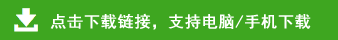# 新人教版小学四年级数学上册竖式、脱式、列式计算题

四年级数学上册竖式、脱式、列式计算题

346+127=   509-261=   729-315=   214+145=   245+136=   756-154=

645-216=   213+237=   900-276=   178+237=   800-216=   900-337=

513-237=   340+216=   200+215=   171+415=   954-327=   270-235=

217+163=   273-136=   498-136=   294+235=   210+337=   152-136=

466+276=   917-119=   437+136=   325-235=   579+235=   662-216=